Next: Calculation of the Core Radius Up: Analysis Previous: Model for the Internal Field Distribution

# Fitting Software

The computer code for fitting the internal field model to the measured muon spin polarisation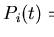is written in FORTRAN. The software incorporates Minuit function minimisation and error analysis routines from the European Organisation for Nuclear Research (CERN) program library. Using trial parameter values, the fitting program calculates the distribution n(f) of muon precession frequencies f according to the field model, and then from n(f) evaluates the corresponding polarisation function (5.1). The code then computes the chi-squared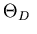between this function and the experimentally recorded muon spin polarisation. Minuit selects new trial values for the variable parameters until it minimises. The fitting program evolved from an earlier version  to include the nonlocal corrections outlined in the previous section and a square vortex lattice geometry.

The fitting program determines the model distribution n(f) of muon frequencies f by sampling the internal field B on a grid of evenly spaced points within the vortex lattice primitive cell. Previous observations (see Chapter 3) indicate that LuNi2B2C has a square vortex lattice at the applied field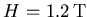of the data analysed in this thesis. Therefore the muon frequency distribution n(f) calculation employs the vortex and reciprocal lattice vector geometry appropriate to a square lattice under an applied field H aligned with the crystalline c axis. In this case the vortex lattice primitive axes b1 and b2 are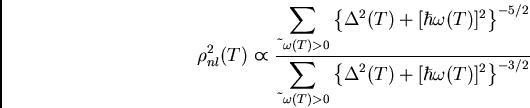where the intervortex spacing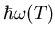. Figure 5.2 shows the sampled locations inside the vortex lattice unit cell.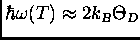The square symmetry of the vortex lattice means the fitting software only needs to sample one eighth of the unit cell. Then, when evaluating the polarisation (5.1), the program weights contributions from locations on the edge of the sampled area by a compensatory factor of one half. Similar symmetry considerations in reciprocal space greatly shorten the time required to compute the field (5.2), and hence dramatically speed up the fitting process. The analysis utilises a sampling density of 5184 points per vortex lattice primitive cell. This density is sufficient since halving it only produces small changes (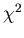) in the best-fit values of the penetration depth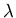and the nonlocality parameter C, and in the calculated core radius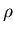.

The ten fitted parameters are

• the average muon precession frequency of the superconducting signal,
• the penetration depth,
• the nonlocality parameter C,
• the effective coherence length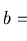,
• the initial amplitude of the asymmetry of the superconducting signal A,
• the initial phase angle of the superconducting signal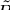,
• the effective depolarisation rate of the the superconducting signal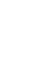due to nuclear dipoles and vortex lattice disorder,
• the average muon precession frequency of the background signal fb,
• the initial phase angle of the background signal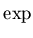, and
• the effective depolarisation rate of the background signal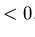.
The data fitting proceeds by alternately letting only the superconducting and then only the background signal parameters vary, until all the variables converge to the final result. Allowing the initial phasesandof the superconducting and background signals to vary separately significantly improves fit quality. During early fitting attempts the initial background asymmetry Ab also varied and grew unphysically large. Therefore throughout all subsequent fits the initial background asymmetry Ab is fixed to 0.0044, as estimated by comparing fast Fourier transforms (FFT) of the polarisation signals measured in the superconducting and normal states. In comparison, the asymmetry A of the superconducting signal is much greater at A = 0.237(2). The fitting takes place within a rotating reference frame set to the expected background signal frequency fb, facilitating easy visual inspection of the accuracy of a fit. Knowledge of the best-fit values for the internal field model parameters enables the extraction of the vortex core radius.Next: Calculation of the Core Radius Up: Analysis Previous: Model for the Internal Field Distribution
Jess H. Brewer
2001-10-31